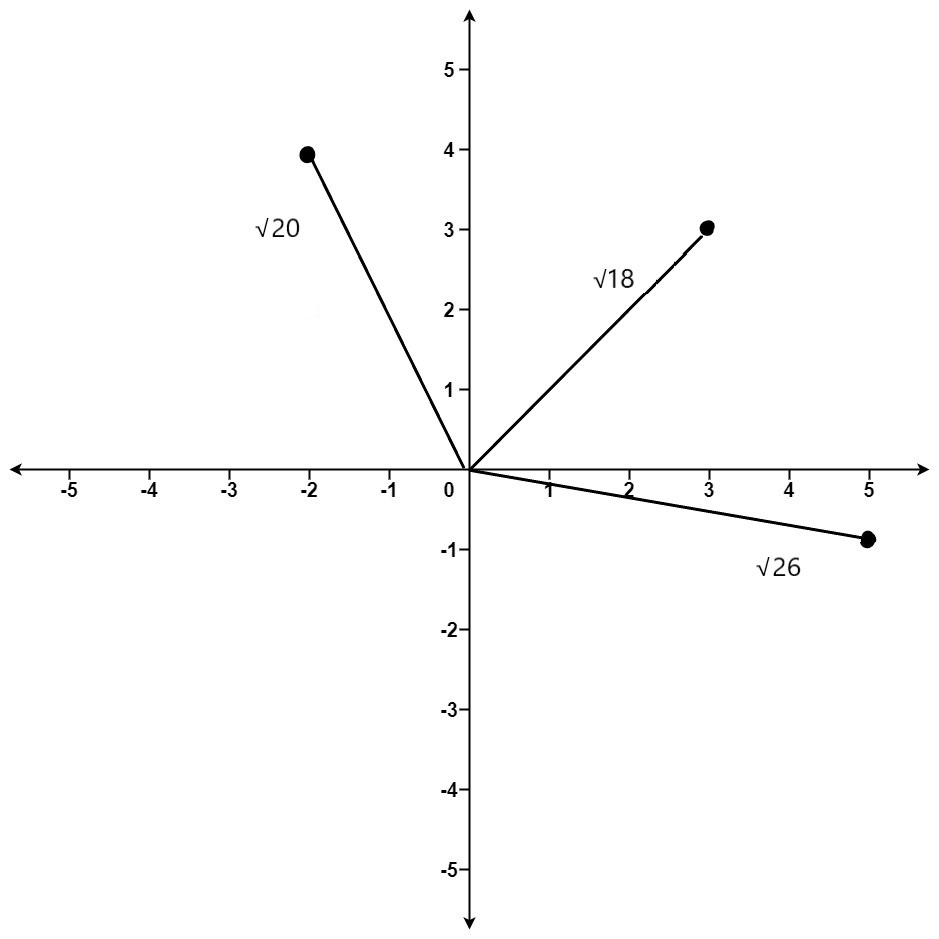# K Closest Points To Origin

## Introduction

K Closest Points To Origin is a simple problem that can be solved using the brute force approach. Here we will discuss the approach and complexity of the algorithm.

## Problem Statement

Given an array of points where points[i] = [xi, yi] represents a point on the X-Y plane and an integer k, return the k closest points to the origin (0, 0).

The distance between two points on the X-Y plane is the Euclidean distance (i.e., √(x1 - x2)^2 + (y1 - y2)^2).

Input:

points = [ [3,3],[5,-1],[-2,4]],

k = 2

Output: [[3,3],[-2,4]]    (these are the 2 closest points among 3 points)

See the below diagram for a better understanding.Note: Please try to solve the problem first and then see the below solution approach.

## Method

Here we will take nothing but a brute force approach to solve this problem. First, we will iterate through all the points and find the corresponding distances from the origin for each point. Then we will find k shortest distances and corresponding points.

For finding the k shortest distances, we need to store the distance and index of the corresponding point. Then we sort the distances and print the first k points corresponding to distances.

## Algorithm

• Iterate over the array of points.
• Find the Euclidean distance of each point from the origin.
• Use a hashmap to store the distance and corresponding point’s index
• Sort the hashmap according to the distances
• Print the k points using the first k indices in the hash map. These are the closest points to origin.

## Code

Output

``````(3,3)
(-2,4)``````

## Complexity analysis

Here the time complexity of the algorithm for finding k closest points is O(nlog n) where n is the number of points.

Space complexity is O(n) as we are using extra space for a hash map.

## FAQs

1). What is Euclidean distance?

Euclidean distance between two points (x1,y1) and (x2,y2) is

√(x1 - x2)^2 + (y1 - y2)^2)

2). What is a hash map?

It is a special kind of data structure that is used to store a unique key and associated value(key-value pair).

3). What kind of sorting is used in the C++ STL sort() function?

It uses a hybrid sorting algorithm of insertion sort, heap sort, quicksort.

## Key Takeaways

Apart from this, you can practice a wide range of coding questions commonly asked in interviews in CodeStudio. Along with coding questions, we can also find the interview experience of scholars working in renowned product-based companies here.

Happy learning!